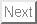Academic Paper
Yuu Miino, Tetsushi Ueta, Hiroshi Kawakami, Nonlinear resonance and devil's staircase in a forced planer system containing a piecewise linear hysteresis, Elsevier, Chaos, Solitons & Fractals, Vol.111, 75-85, June 2018.
Abstract: The Duffing equation describes a periodically forced oscillator model with a nonlinear elasticity. In its circuitry, a saturable-iron core often exhibits a hysteresis. However, a few studies about the Duffing equation has discussed the effects of the hysteresis because of difficulties in their mathematical treatment. In this paper, we investigatea forced planer system demonstrating the Duffing equation containing the hysteresis. For simplicity, we approximate the hysteresis to a piecewise linear function. Since the solutions are expressed by combinations of some dynamical systems and switching conditions, a finite-state machine is derived from the hybrid system approach, and then bifurcation theory can be applied to it. We topologically classify periodic solutions and compute local and grazing bifurcation sets accurately. In comparison with the Duffing equation, we discuss the effects caused by the hysteresis, such as the devil's staircase in resonant solutions.

Contact address: ueta @ tokushima-u.ac.jp
2020-10-01